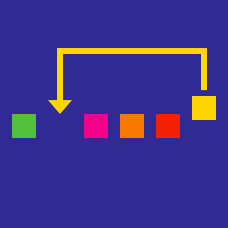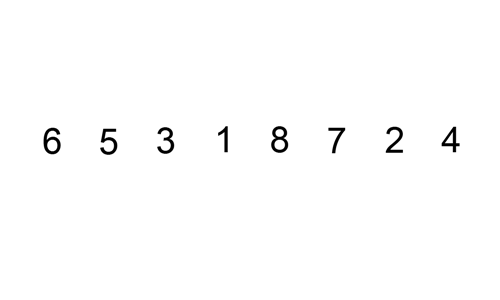Computer Science

# Sorting Algorithms: Level 3 challenges

Consider the modification of a 4 way merge sort which instead of dividing an array into two subarrays, 4-way merge sort divides the array into four sub-arrays and sorts each individual array recursively.

In the 2-way merge sort we have an index for each of the two sorted sub-arrays and we compare the elements they are pointing to and in worst case we perform $2k-1$ comparison where $k$ is the length of each array. Similarly in a $4$-way merge sort each of size $k$ we have and index for each of the four arrays. It takes $3$ comparisons to determine the smallest of the four. In worst case we must do this until each list has one element left for a total of $12(k-1)$ comparison. Finally we perform $3+2+1$ comparisons to finish the remaining list, thus for a total of $12k-6$ comparisons.

Based on the above merge procedure which of the following represents the correct running time for a $4$ way merge sort?

Details and Assumptions

-Ignore constant terms.

Is it possible to come up with a better worst case running time? is it asymptotically better?

Suppose you are give $k$ sorted arrays each of $n$ elements. Using the standard merge subroutine you merge the first 2 arrays and merge the 3rd array to the already merged arrays, and so on until you merge the $k$-th array, what is the running time of this procedure?We know that the insertion sort algorithm has a worst-case running time of $O(n^{2})$ in general case. What if we could bound the algorithm for specific instance of the problem. One way to do that is by checking the number of swap operations needed by insertion algorithm to sort a list.

In the permutation of $a_{1}\cdots a_{n}$ of $n$ distinct elements the list inversion are pair of elements $(a_{i},a_{j})$ such that $i and $a_{i} > a_{j}$. What is the worst-case running time if the inputs are restricted to permutations of $1\cdots n$ with at most $n$ inversions?

×